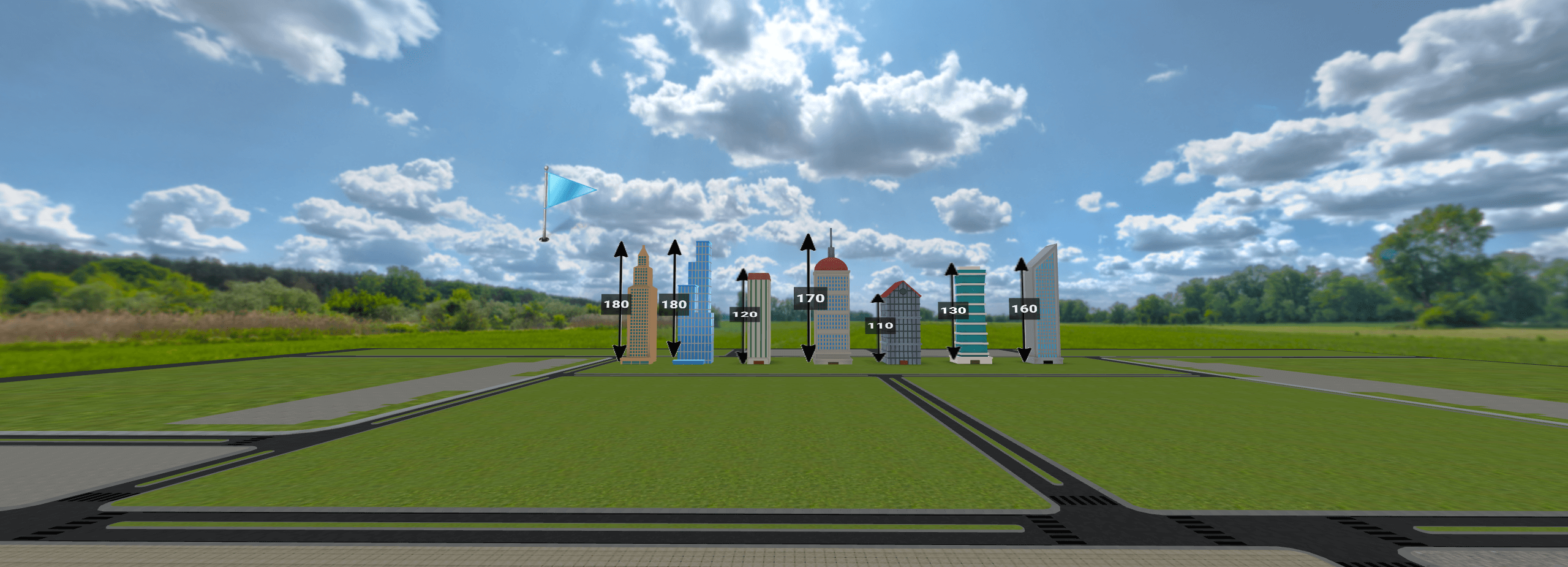Connect with our sales team.

#### Get Started

Median, Mode, Mean & Range

LaunchSubject: Math

Mean is defined as the average of a set of numbers that can be calculated by adding all the numbers and dividing the sum by the total number of terms. Median is defined as the middle value in a given set of numbers. To find the median, list all the numbers in increasing or decreasing order. The mode is the value that occurs most frequently in a given set of numbers. Range is the difference between the lowest and highest values in a given set of numbers.

Ready to transform your classroom with immersive learning technology? Connect with our sales team.

#### Get Started

Median, Mode, Mean & Range

Launch

Subject: Math